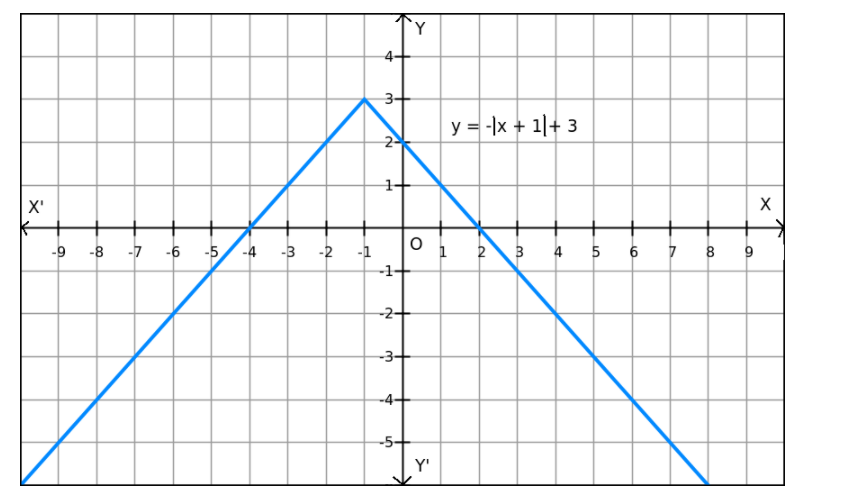# If the solve the problem`
Question:

$f(x)=-|x+1|+3$ on $R$

Solution:

Given: $f(x)=-|x+1|+3$

Now,

$-|x+1| \leq 0$ for all $x \in R$

$\Rightarrow f(x)=-|x+1|+3 \leq 3$ for all $x \in R$

$\Rightarrow f(x) \leq 3$ for all $x \in R$The maximum value of $f$ is attained when $|x+1|=0$.

$\Rightarrow x=-1$

Therefore, the maximum value of $f$ at $x=-1$ is 3 .

Since f(x) can be reduced, the minimum value does not exist, which is evident in the graph also.
Hence, the function f does not have a minimum value.